### Project Cost Estimation Template In Excel

Every project manager has to take care about cost of project. Use this to calculate it within Excel.

1. Enter data into Excel regarding project cost.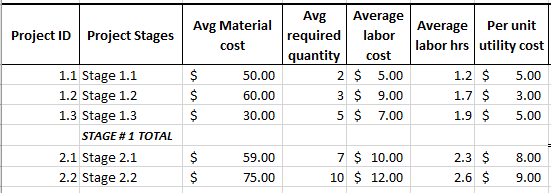2. Calculate total of each stage by using sum formula. Then drag it horizontally to get the sum of other columns.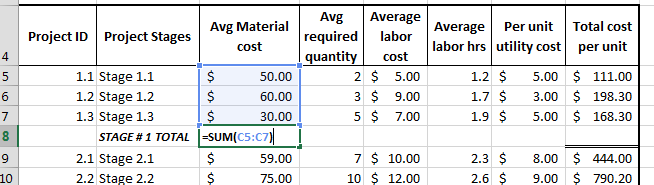3. Revise step two for stage 2 total.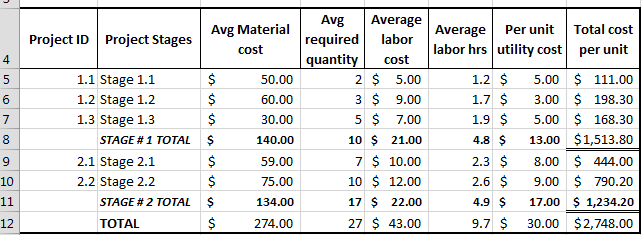4. Now, add another column of total cost per unit, which includes all horizontal additions.5. Make two more rows below this table: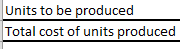6. Enter the no of units in the cell corresponding to units to be produced. Then, multiply units to be produced by total cost per units (both highlighted in the red in the following screen shot). This is your total cost of production.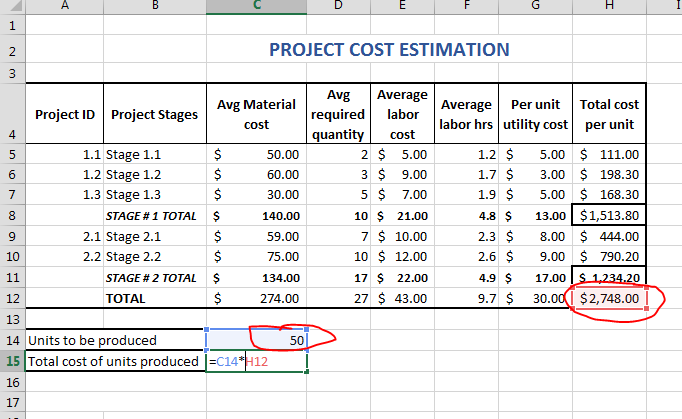This is how to estimate project costs in Excel.

## Template

```Further reading: